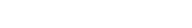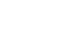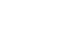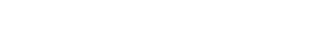# Earth Pressure Method For Retaining WallsReading time: 1 minute

Earth Pressure Method For Retaining Walls

Cantilever wallsWhen designing sheet retaining walls it is normal to assume that the effective lateral stresses acting on the wall are given by simple RANKINE active and passive zones.  Friction on the wall is usually ignored as this leads to conservative (safe) designs.

Rankine Active and Passive Pressures

The earth pressures acting on the wall are strongly dependent on the deformations in the surrounding soil.  When the wall moves away from the soil the stress on the wall drops reaching a minimum, the ACTIVE pressure, with the soil deforming plastically.  When the wall moves into the soil the stress increases, finally reaching a maximum, the PASSIVE pressure, when again the soil is deforming plastically.For most retaining walls the long term, fully drained, situation usually governs the wall stability. For the analysis of fully drained conditions the Mohr-Coulomb criterion needs to be expressed in terms of effective stress using the effective strength parameters c´ and ?´. For design it is also conservative to use the critical state strength parameters, that is c´ = 0 and ?’ = ?’v. The effective lateral stresses on the wall are thenKa and Kp are known as the active and passive earth pressure coefficients. For soil at failure the earth pressure coefficients are simply related by.

For any vertical wall it is possible to relate the horizontal effective stress to the vertical effective stress, determined from the vertical overburden, by an earth pressure coefficient. The coefficient will depend on the slope of the soil surface and the wall roughness. Published values are available for many situations.

Stability - Limiting Equilibrium

When assessing the stability it is normal to assume triangular pressure distributions, and this is in fact quite realistic if the wall is rigid.  For a cantilever wall the stresses acting at failure will then be as shown below, with the wall rotating about a point just above the toe of the wall.  The stability of the wall depends mainly on the passive force developed below the excavation.For design we need to determine the required depth of penetration for stability and then to size the wall to resist the maximum moment.  To determine the depth of penetration required for a given height H we need to consider both moment and force equilibrium:

? F = 0

? M = 0

If the soil is dry the pressures and forces are as shown belowWe have 2 equations with 2 unknowns, x and d, and hence we can determine the required depth of penetration for the wall. The equations can be solved graphically or by computer. Alternatively simplifying assumptions about the forces below the pivot can be made to enable analytical solutions to be obtained as described in many text books.Serviceability - Design requirements

By considering the stability we can obtain the limiting stresses on the wall, but the wall would have been considered to have failed from a serviceability viewpoint well before this, owing to large settlements in the supported soil.  The design approach is to factor the earth pressures.

There are two main design approaches which are both based on the knowledge that the earth pressures acting on the wall are strongly dependent on the deformations in the surrounding soil. The movements required to reach the active and passive conditions depend on the soil type and can be quite different. For example, for retaining walls of height H the movements required are approximately:

 Sand Active 0.001H Passive 0.05H – 0.1H Clay Normally Consolidated Active 0.004H Passive Large Over- Consolidated Active 0.025H Passive 0.025H

Method 1 - Sands and normally consolidated clay

Assume that sufficient movement occurs to allow active (minimum) pressures to develop, and then factor the effective passive pressures by 2.  Note that where insufficient movement of the wall occurs the active pressures will not reach minimum and higher pressures will act on the wall. These must be allowed for in design as they can influence the required structural strength.

Consider the same wall as above with H = 1.8, ?d = 19 kN/m3, ?´ = 30o

The pressure diagram looks identical but the passive pressures are reduced by using a reduced value of the passive earth pressure coefficient, K*p.

Where K*p = Kp/2  =  1.5  and K*a = Ka = 0.3333 as before.

Hence d = 2.94 m

The total depth of sheet pile required = 1.8 + 2.94 = 4.74 m

Some texts recommend increasing the depth of penetration by a further 10-20% to allow for uncertainties in the analysis. Alternatively some design codes recommend assuming the top 0.5 m of the soil beneath the excavation provides no restraining effect.

Method 2 - Over-consolidated clays

Here both active and passive pressures are developed for similar movements and both are factored.  This is achieved by dividing tan?’ by a Factor F?, and using the reduced angle of friction when calculating the earth pressure coefficients K*a, K*p.  The factor F? can be in the range from 1.2 to 1.5, depending on the allowable settlement and soil type, but is usually taken as 1.3.

As for method 1 it is assumed that the shape of the pressure diagram is similar to that at limiting equilibrium, but in this case the passive pressures are reduced and the active pressures increased.

Structural strength

Having determined the required depth of penetration, the next stage in design is to calculate the maximum moment in the wall so that an appropriate wall thickness and strength can be selected. The position down the wall of the maximum moment can be found by determining where the shear stress in the wall is zero. ()

Consider a free body diagram of a section of the wallA quadratic equation that can be solved for z using appropriate (factored) values for Kp, Ka.

Then taking momentsNote that as the factor of safety increases the maximum moment also increases.

The factor of safety can be dramatically reduced by surcharge loadings on the supported ground next to the wall.  For a uniform surcharge then the effective active pressure can be increased by Ka ss, while for a concentrated load from a footing the Coulomb method of trial wedges can be used to determine the active force on the wall.  In the latter situation allowance must be made for the fact that the point of application of the load will also change.Consideration must also be given to the water pressures acting on the wall.For economic reasons cantilever walls are usually limited to excavations less than 6 m deep.

They are often used to support low banks of free draining sand and gravel soils.

They are not suitable for the long term support of soft clayey soils (clay or silt)

Corrosion can also be a problem with steel sheet piles.